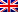# Seminario di Fisica Matematica

Martedì 17 maggio 2022 alle ore 16:30, Hagop Tossounian (CMM, at Universidad de Chile), terrà il Seminario dal titolo "Entropic chaoticity and Fisher information type chaoticity for a family of rescaled states (j. w. Roberto Cortez)".

Abstract:
For a probability measure $f$, and each $N >=2$ we introduce an exchangeable random variable obtained from rescaling Y (Law(Y)= $f^{\otimes N}$) to the sphere $\sum {x_j}^2 = N$. It is known  that all the k-marginals of these processes converge weakly to $f^{\otimes k}$,(a property known as chaoticity and used by Mark Kac ). The aim of the talk is to show that the chaos property of this sequence of rescaled r.v. can be strengthened to entropic chaos and to
Fisher-information chaos, under mild assumptions on $f$. This work is
j.w. Roberto Cortez arXiv:2204.05406.

 Kac M.Foundations of Kinetic Theory. Proceedings of the Third Berkeley Symposium on Mathematical Statistics and Probability, 1954 − 1955, vol. III, pp. 171–197. University of California Press, Berkeley and Los Angeles, 1956.

 Cortez, R., Tossounian, H. On a Thermostated Kac Model with Rescaling.
Ann. Henri Poincaré 22, 1629–1668 (2021).

Il seminario avrà luogo in presenza presso il Dipartimento di Matematica e Fisica, L.go S. L. Murialdo 1 - Aula 211
This post is also available in: Link identifier #identifier__16147-5Eng